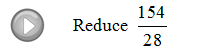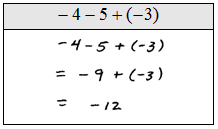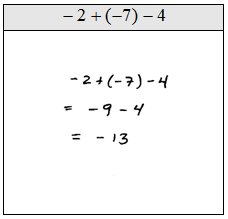## Algebra

Showing posts with label operations. Show all posts
Showing posts with label operations. Show all posts

### Fractions

Fractions can be a barrier to beginning algebra students.  Also referred to as rational numbers, fractions are simply real numbers that can be written as a quotient, or ratio, of two integers.Equivalent fractions can be expressed with different numerators and denominators. For example,If you eat 4 out of 8 slices of pizza, that is the same as eating one-half of the pie.  Usually we will be required to reduce fractions to lowest terms. Fractions in lowest terms have no common factors in the numerator and denominator other than 1.An alternative and more common method of reducing is to identify the greatest common factor (GCF) of the numerator and denominator and then divide both by that number.An improper fraction is one where the numerator is larger than the denominator.  To convert an improper fraction to a mixed number, simply divide. The quotient is the whole number part and the remainder is the new numerator.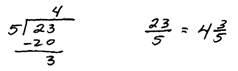Reduce.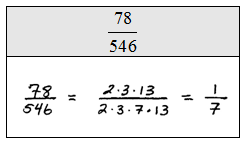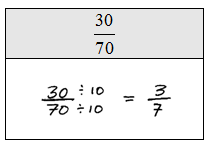To multiply fractions, you multiply the numerators and the denominators. NO COMMON DENOMINATOR is required.To divide fractions, you multiply the numerator by the reciprocal of the divisor.When multiplying, look for factors to cancel before actually multiplying the numerators and denominators.  This will eliminate the need to reduce the end result.

Multiply or Divide.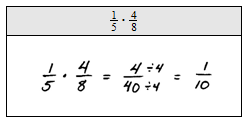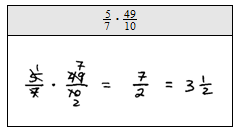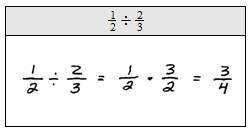Convert mixed numbers to improper fractions before you multiply or divide. To do this, multiply the denominator with the whole number then add the numerator, this will be the new numerator.Divide.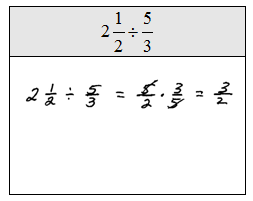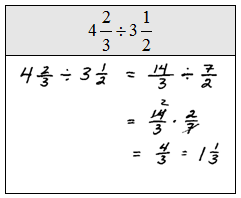Sometimes mixed numbers are confused with multiplication.  Be sure to remember that 4 2/3 does not imply multiplication, it represents addition.The difficulty with fractions is usually caused by addition and subtraction. These operations require a common denominator.  If I were to say that I ate two pieces of pizza, you would want to know what size each piece was.  To say that I ate 2 pieces out of a pizza cut into 4 big slices is not the same as eating 2 pieces of a pizza cut into 8 slices!

If fractions have a common denominator, simply add or subtract the values found in the numerator and write the result over the common denominator and reduce if necessary.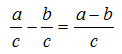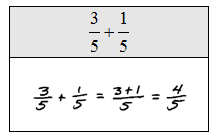Many of the problems that you are likely to encounter will have different denominators. You will have to find the equivalent fractions with a common denominator before you can add or subtract them.

Step 1: Determine the least common multiple of the denominators (LCD).
Step 2: Multiply numerator and denominator by what you need to obtain equivalent fractions with that LCD.
Step 3: Add the numerators and write the result over the common denominator.
Step 4: Reduce if necessary.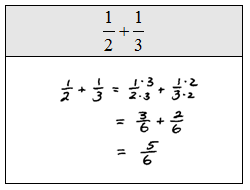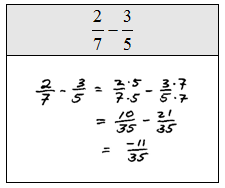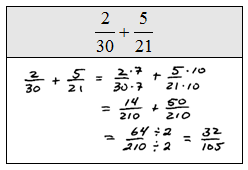Division Word Problem: A board that is 5 1/4 feet long is cut into 7 pieces of equal length.  What is the length of each piece?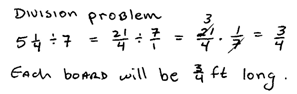Multiplication Word Problem: A muffin recipe calls for 1 2/3 cups of flour to make 4 muffins.  How many cups of flower does the recipe require if you wish to make 16 muffins?